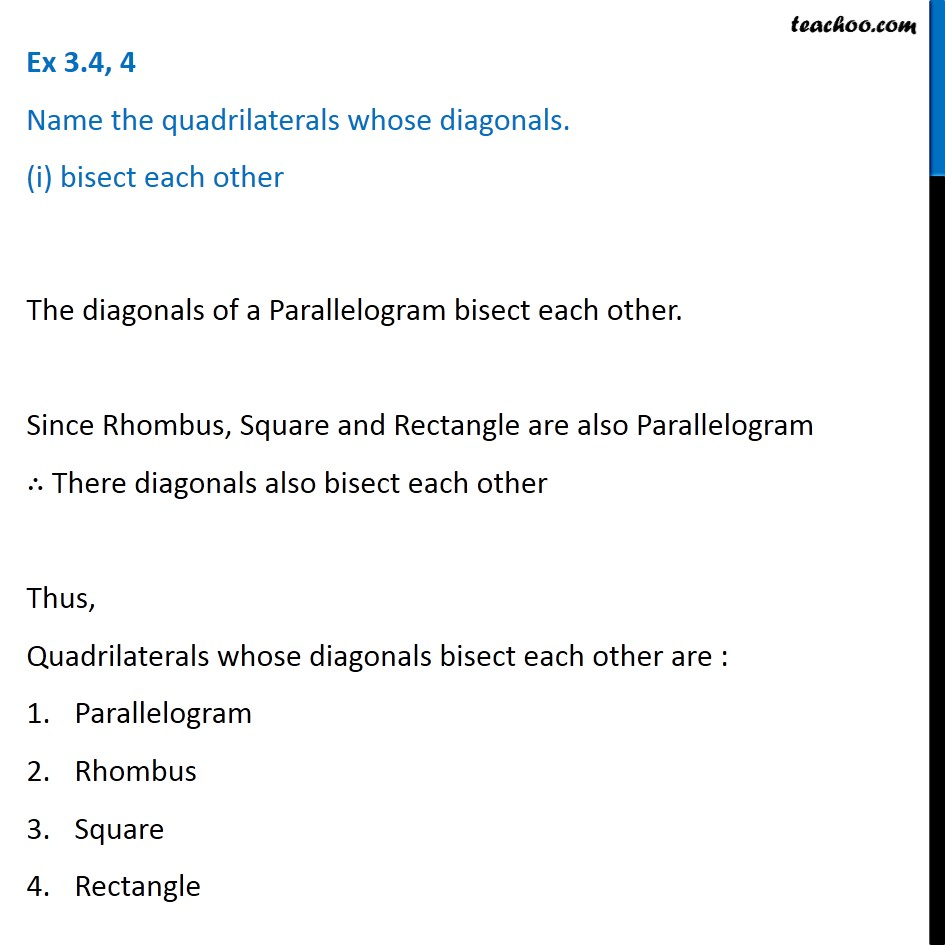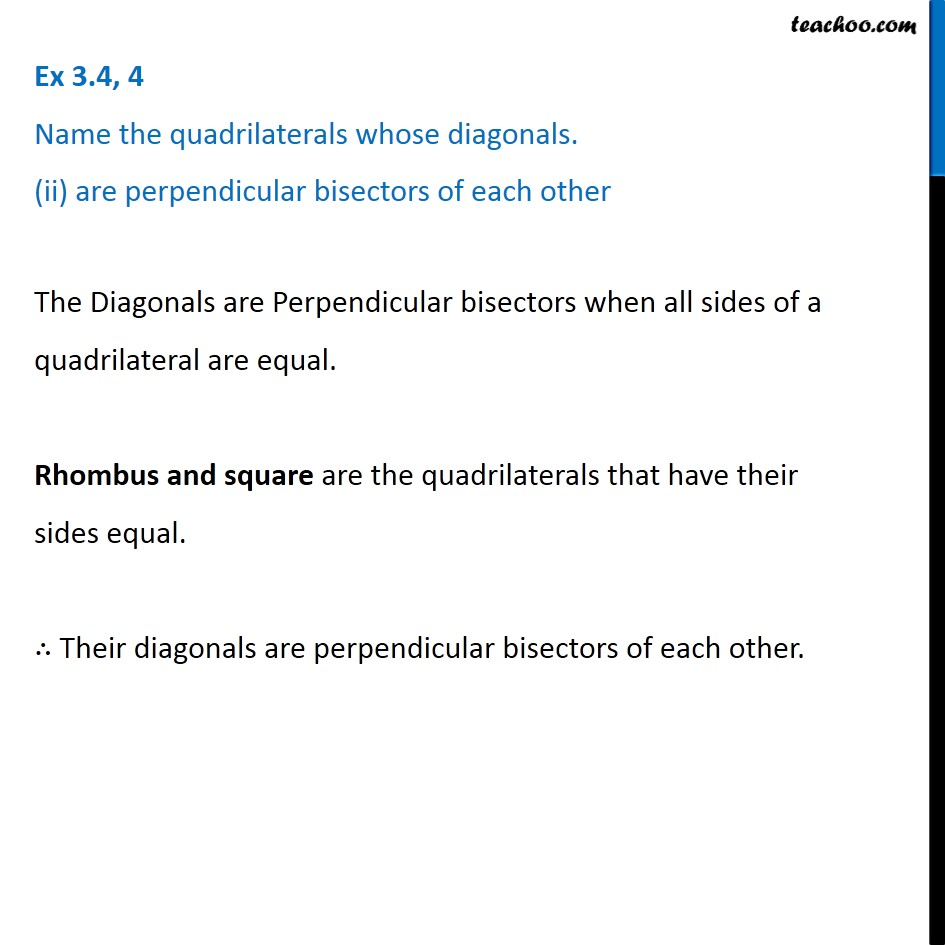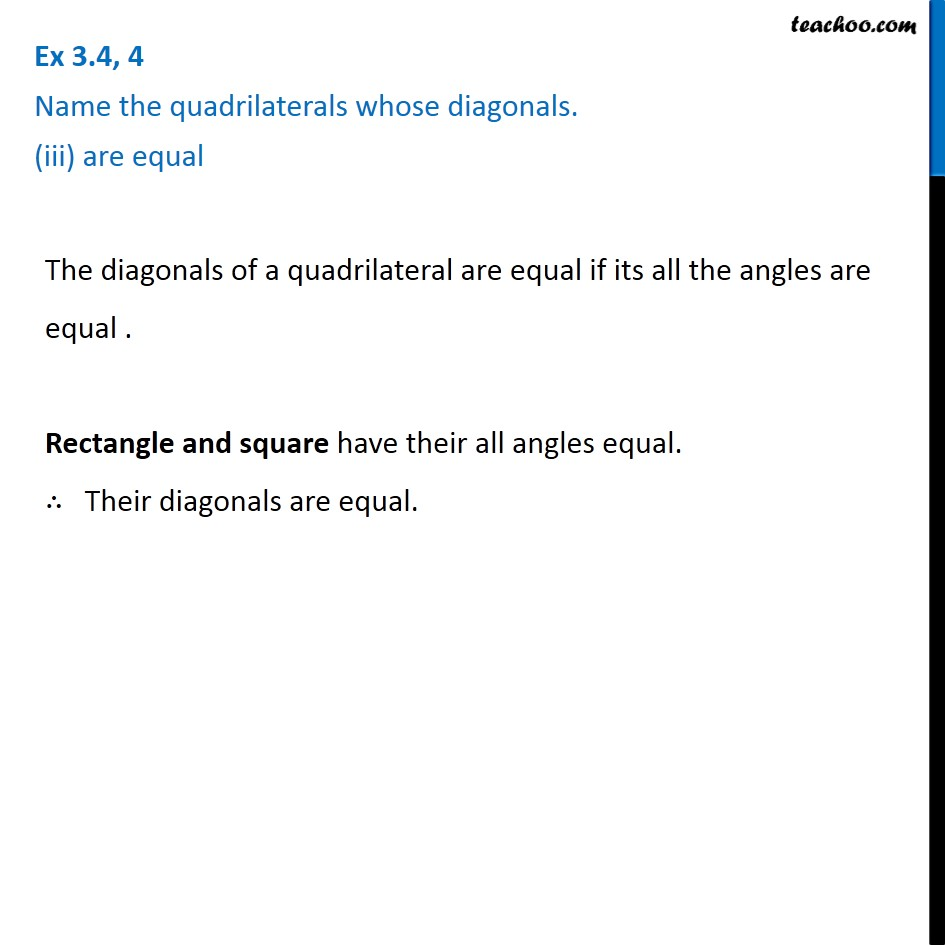Subscribe to our Youtube Channel - https://you.tube/teachoo

1. Chapter 3 Class 8 Understanding Quadrilaterals
2. Serial order wise
3. Ex 3.4

Transcript

Ex 3.4, 4 Name the quadrilaterals whose diagonals. (i) bisect each other The diagonals of a Parallelogram bisect each other. Since Rhombus, Square and Rectangle are also Parallelogram ∴ There diagonals also bisect each other Thus, Quadrilaterals whose diagonals bisect each other are : Parallelogram Rhombus Square Rectangle Ex 3.4, 4 Name the quadrilaterals whose diagonals. (ii) are perpendicular bisectors of each other The Diagonals are Perpendicular bisectors when all sides of a quadrilateral are equal. Rhombus and square are the quadrilaterals that have their sides equal. ∴ Their diagonals are perpendicular bisectors of each other. Ex 3.4, 4 Name the quadrilaterals whose diagonals. (iii) are equal The diagonals of a quadrilateral are equal if its all the angles are equal . Rectangle and square have their all angles equal. ∴ Their diagonals are equal.

Ex 3.4